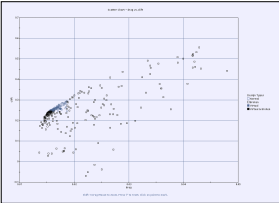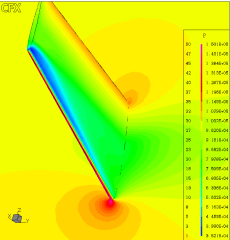# Transonic 3D Wing Multi-Objective Optimization

Author(s):
Clarich, Poloni (Universita' degli studi di Trieste)

The purpose of this work is the optimization of the ONERA M6 wing in order to reduce the inviscid drag with constraints on the lift coefficient and on the maximum thickness of spanwise wing cuts. The lift coefficient should be greater than or equal to the coefficient calculated for the original wing geometry, while the maximum thickness of the wing should be the same of the original geometry.  For the simulation of the flow we have used a 3-D parametric structured mesh, while the flow solutor employed is CFX-Tascflow, a numeric code based on Navier-Stokes equations. These software platforms were coupled with modeFRONTIER, which performed the optimization through a multi-objective genetic algorithm, with penalizing functions for the constraints. The objectives were the minimization of the drag and the maximization of the lift, with a constraint on the drag that should be less than the original one and on the lift that should be greater than the original one.  In the optimization we have computed a total of 352 designs, divided in 11 generations of 32 individuals each one. A reduced factorial DOE was used. At the end the optimization of the ONERA M6 wing geometry gave two different configurations characterized by a best value of lift and a lower value of drag.